# 黎巴嫩首都爆炸能量有多大？物理学家看视频计算：300吨TNT！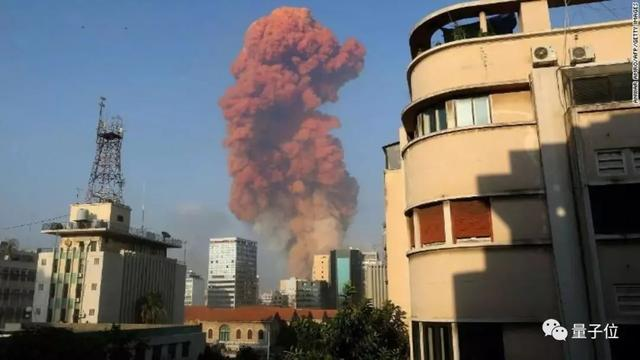# 从照片中窥测核爆机密

1950年，也就是首次核试验“三位一体”之后5年，美国军方公开当时的照片，照片中只标注了比例尺和时间。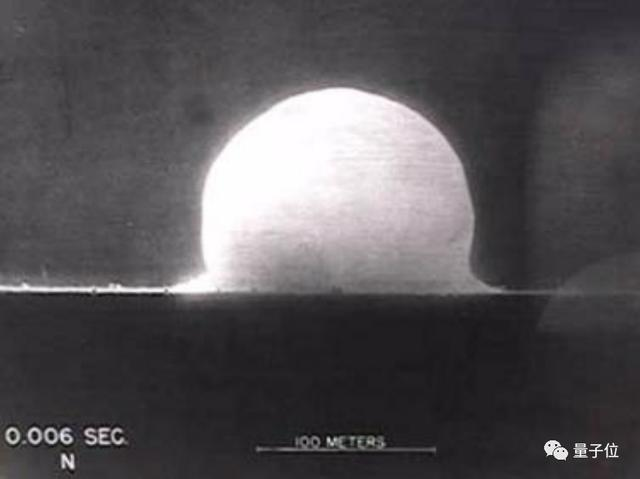R：爆炸冲击波球的半径；

E：爆炸释放的能量；

ρ：爆炸冲击波传播介质的密度；

t：距离起爆的时间。# 估算贝鲁特港爆炸威力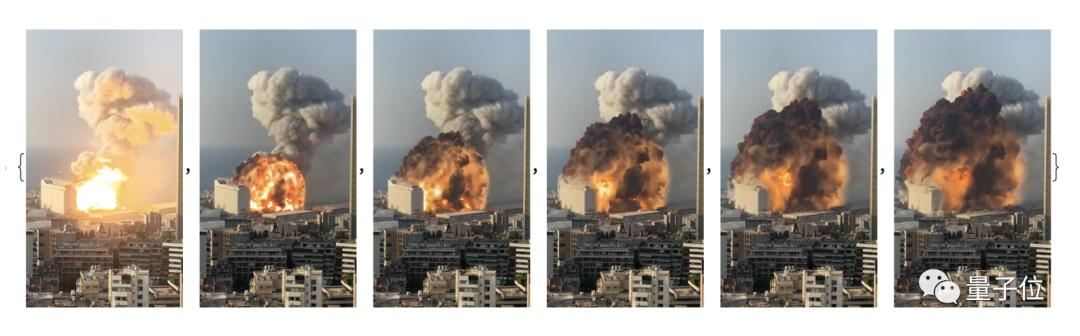``````circs = CircleThrough /@ data[[;; 6, 2]];
r = circs[[All, 2]];``````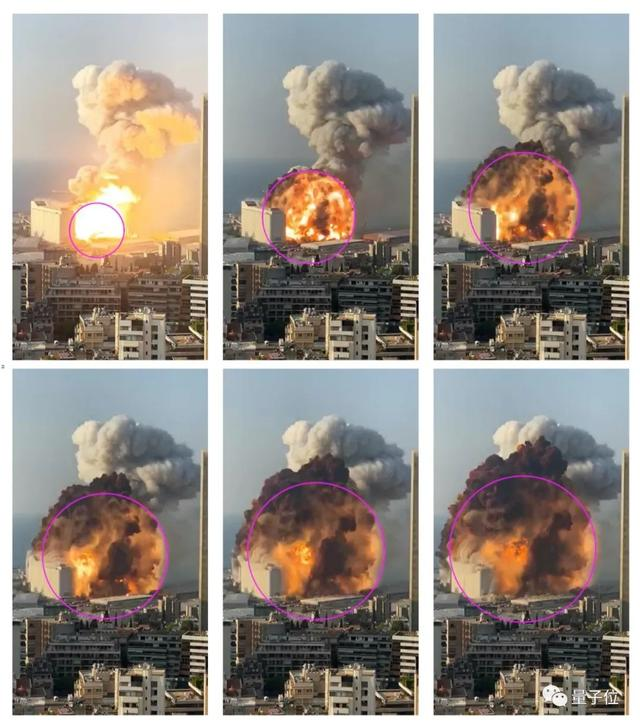``````cali = 0.5888486673789164`;
realr = r cali``````

``````fit = FindFit[
tr, { a (x + t0)^0.4, 0 < t0 < 1/30}, {{a, 200}, {t0, 1/60}}, x]
realfit = a (x + t0)^0.4 /. fit
tzero = t0 /. fit
realfitshifted = a (x)^0.4 /. fit
prefactor = a /. fit``````

``````Show[{ListPlot[Transpose[{t + tzero, realr}]],
Plot[realfitshifted, {x, 0, 0.2}]},
PlotRange -> {{0, 0.2}, {0, 120}}, Frame -> True,
FrameLabel -> {"t", "r [m]"}]``````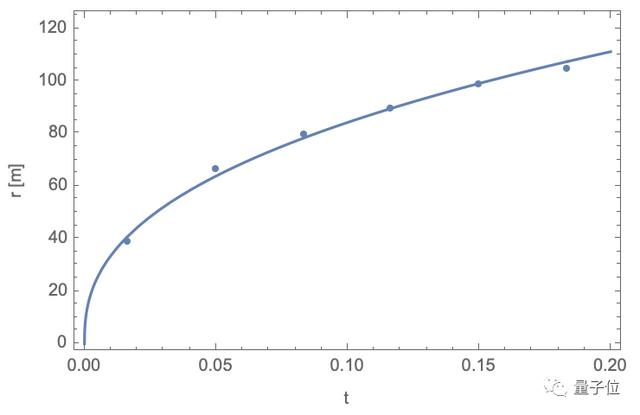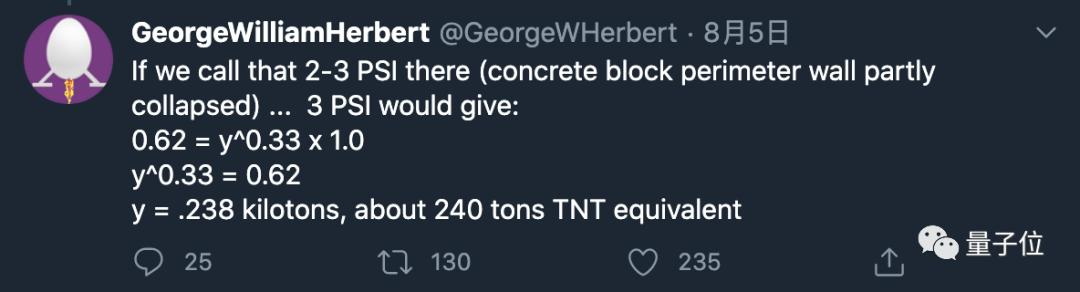http://sites.science.oregonstate.edu/~restrepo/MTH481/Classnotes/GITaylor/GITaylorAnalysis.pdf

https://community.wolfram.com/groups/-/m/t/2051264